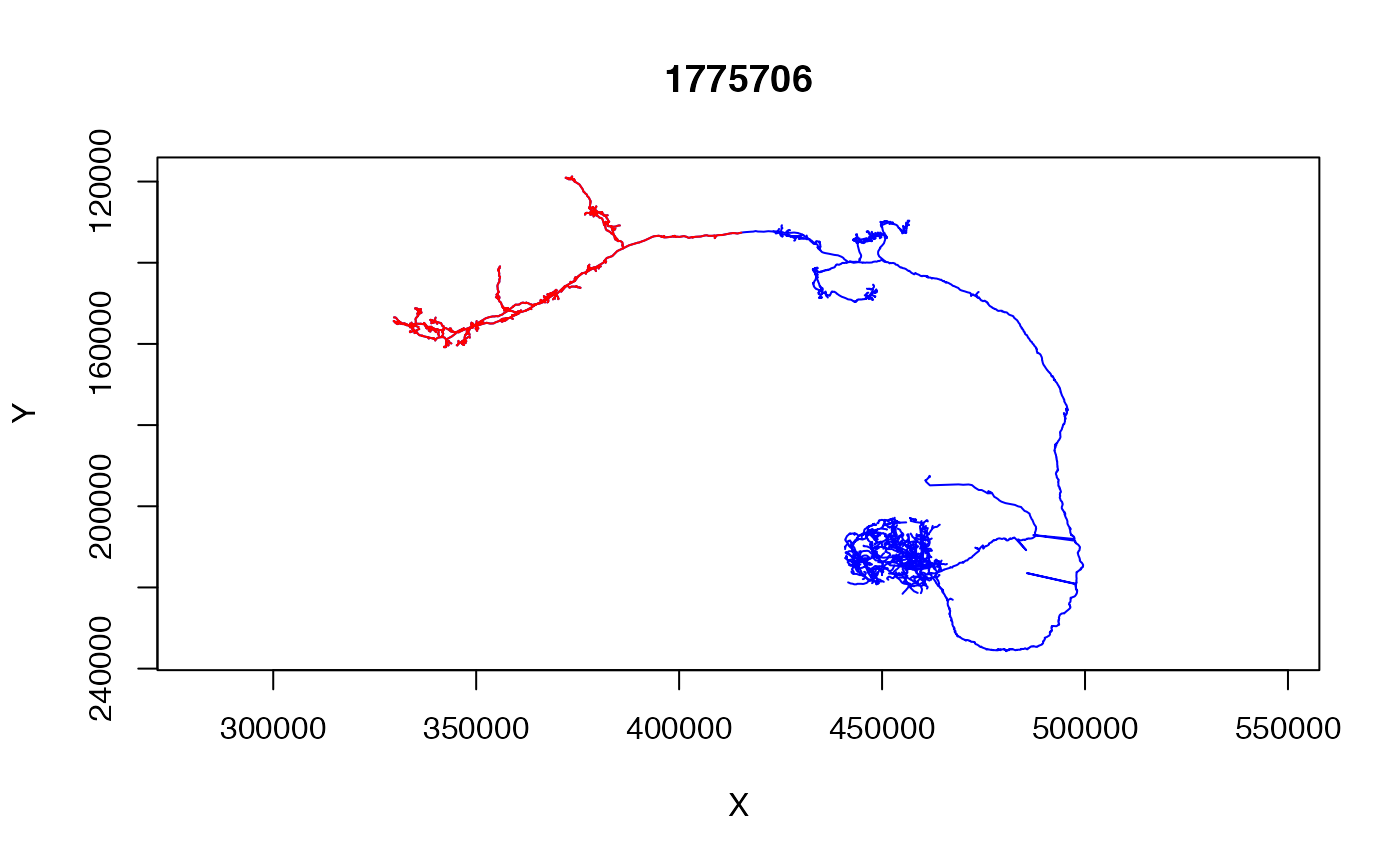This function returns a list (containing the order of nodes) travelled using a depth first search starting from the given node.

distal_to(
x,
node.idx = NULL,
node.pointno = NULL,
root.idx = NULL,
root.pointno = NULL
)

## Arguments

x A neuron The id(s) of node(s) from which distal points will be selected. node.idx defines the integer index (counting from 1) into the neuron's point array whereas node.pointno matches the PointNo column which will be the CATMAID id for a node. The root node of the neuron for the purpose of selection. You will rarely need to change this from the default value. See node argument for the difference between root.idx and root.pointno forms.

## Value

Integer 1-based indices into the point array of points that are distal to the specified node(s) when traversing the neuron from the root to that node. Will be a vector if only one node is specified, otherwise a list is returned

subset.neuron, prune

## Examples

# \donttest{
## Use EM  finished DL1 projection neuron

## subset to part of neuron distal to a tag "SCHLEGEL_LH"
# nb distal_to can accept either the PointNo vertex id or raw index as a
# pivot point
dl1.lh=subset(dl1neuron, distal_to(dl1neuron,
node.pointno = dl1neuron$tags$SCHLEGEL_LH))
plot(dl1neuron,col='blue', WithNodes = FALSE)
plot(dl1.lh, col='red', WithNodes = FALSE, add=TRUE)# }